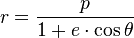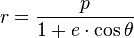# Mathematical Proof of Kepler's First Law of Orbits

• B
Memocyl
Hello friends (I hope),

For a maths project I am working on, I need to be able to prove the equation for an elliptical orbit, related to Kepler's first law:and p = a(1-e2) (or should be as p can be replaced by that value)

Where:
r = distance from sun to any point on the orbit
p = semi latus rectrum
a = semi-major axis
e = eccentricity
θ = true anomaly (angle between a and r anticlockwise I think)

Can someone please help me to understand where these equations come from and also confirm that I have got my current facts straight?

Regards,
Memocyl

Staff Emeritus
Gold Member
Have you looked in classical mechanics and/or astrophysics books?

Memocyl
Have you looked in classical mechanics and/or astrophysics books?
I haven't been able to come by any at the moment that help me derive the equation itself, which is what I don't understand. However, if you could name me any books that do that would be exceptionally helpfulThank you
Memocyl

Staff Emeritus
Gold Member
Examples at the level of about second-year university include "Analytical Mechanics" by Fowles and Cassiday, and "Foundations of Astrophysics" by Ryden and Peterson. I haven't looked, but I suspect that derivations of Kepler's first law also can be found on-line. Just Google "Kepler's first law".

Memocyl
Examples at the level of about second-year university include "Analytical Mechanics" by Fowles and Cassiday, and "Foundations of Astrophysics" by Ryden and Peterson. I haven't looked, but I suspect that derivations of Kepler's first law also can be found on-line. Just Google "Kepler's first law".
I have tried online but to no avail unfortunately, apart from it bringing me here actually. Thank you very much though, I shall look for those books as soon as possible.

Regards
Memocyl

Staff Emeritus
Homework Helper
Hello friends (I hope),

For a maths project I am working on, I need to be able to prove the equation for an elliptical orbit, related to Kepler's first law:and p = a(1-e2) (or should be as p can be replaced by that value)

Where:
r = distance from sun to any point on the orbit
p = semi latus rectrum
a = semi-major axis
e = eccentricity
θ = true anomaly (angle between a and r anticlockwise I think)

Can someone please help me to understand where these equations come from and also confirm that I have got my current facts straight?

Regards,
Memocyl
There's plenty of material online which derives Kepler's laws from different perspectives.

Look at:
https://en.wikipedia.org/wiki/Kepler's_laws_of_planetary_motion (check out the bibliography at the end of the article)

https://en.wikipedia.org/wiki/Ellipse (for details of the equation of the ellipse in polar form)

http://www.grputland.com/2013/12/self-contained-derivation-of-keplers-laws-from-newtons-laws.html